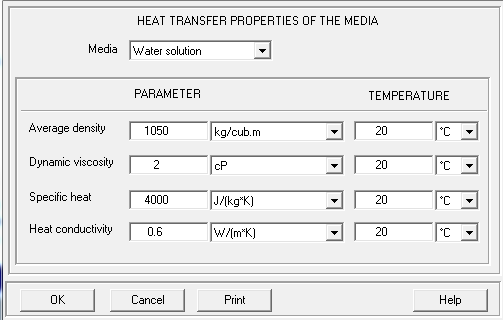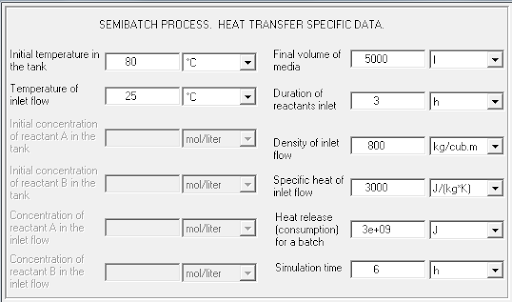In this case, the semi batch process need to be cooling with a liquid heat transfer agent.  No chemical reaction occurs. The lower limit of temperature is 40 C and the upper is 140 C. Subject of calculations: Mixing in a reactor with a 2-stage Lightnin A310.  Additional technical information. Tank:
• Elliptical bottom – conventional jacket
• Inside diameter – 1800 mm
• Total height- 2200 mm
• Volume of media – 5000 liter.
• Material – stainless steel (generalized)
• Thermal resistance of fouling – 0.0002 (m2*K)/W
• Mass – unknown
• Wall thickness – 6 mm.
• Tank head type – Absent
• Jacket (Covers bottom – one section)
• Height, Hlow – 1530 mm
• Heat transfer area – 11.5 m2
• Enhancing device – Absent
• Width – 50 mm
• Wall thickness – 6 mm.
Baffle (Flat, at distance to the tank wall):
• Number = 4
• Width = 160 mm;
• Length = 1700 mm;
• Distance from bottom = 450 mm.
• Distance from the wall = 50 mm
• Angle to radius = 0 deg.
Impeller :
• 2-stage Lightnin A310
• Tip diameter = 1000 mm
• Distance between stages = 850 mm
• Distance from bottom = 400 mm.
• Rotational speed = 100 rpm
• Power of drive = 5 kW
Media properties:
• Density of media – 1050 kg/m3
• Viscosity of media – 2 cP.
Heat Transfer Data:
• Cooling liquid agent – Water
• Inlet temperature = 20 C
• Flow rate = 3.2 m3/hFor this process, we want to determine the following heat transfer parameters:
0%
102

Test 7 – Heat transfer semi batch

In this case, the semi batch process need to be cooling with a liquid heat transfer agent.

No chemical reaction occurs. The lower limit of temperature is 40 C and the upper is 140 C.

Subject of calculations:

Mixing in a reactor with a 2-stage Lightnin A310.

Tank:

• Elliptical bottom – conventional jacket
• Inside diameter – 1800 mm
• Total height- 2200 mm
• Volume of media – 5000 liter.
• Material – stainless steel (generalized)
• Thermal resistance of fouling – 0.0002 (m2*K)/W
• Mass – unknown
• Wall thickness – 6 mm.
• Tank head type – Absent
• Jacket (Covers bottom – one section)
• Height, Hlow – 1530 mm
• Heat transfer area – 11.5 m2
• Enhancing device – Absent
• Width – 50 mm
• Wall thickness – 6 mm.

Baffle (Flat, at distance to the tank wall):

• Number = 4
• Width = 160 mm;
• Length = 1700 mm;
• Distance from bottom = 450 mm.
• Distance from the wall = 50 mm
• Angle to radius = 0 deg.

Impeller :

• 2-stage Lightnin A310
• Tip diameter = 1000 mm
• Distance between stages = 850 mm
• Distance from bottom = 400 mm.
• Rotational speed = 100 rpm
• Power of drive = 5 kW

Media properties:

• Density of media – 1050 kg/m3
• Viscosity of media – 2 cP.

Heat Transfer Data:

• Cooling liquid agent – Water
• Inlet temperature = 20 C
• Flow rate = 3.2 m3/hFor this process, we want to determine the following heat transfer parameters:

Heat Transfer Rate – maximum value reached, approximate = W

The upper temperature limit is reached

Media Temperature: What is the maximum value reached?

Overall heat transfer coefficient: After about 175 min it starts to decrease

Which is its maximum achieved value (approximate)?